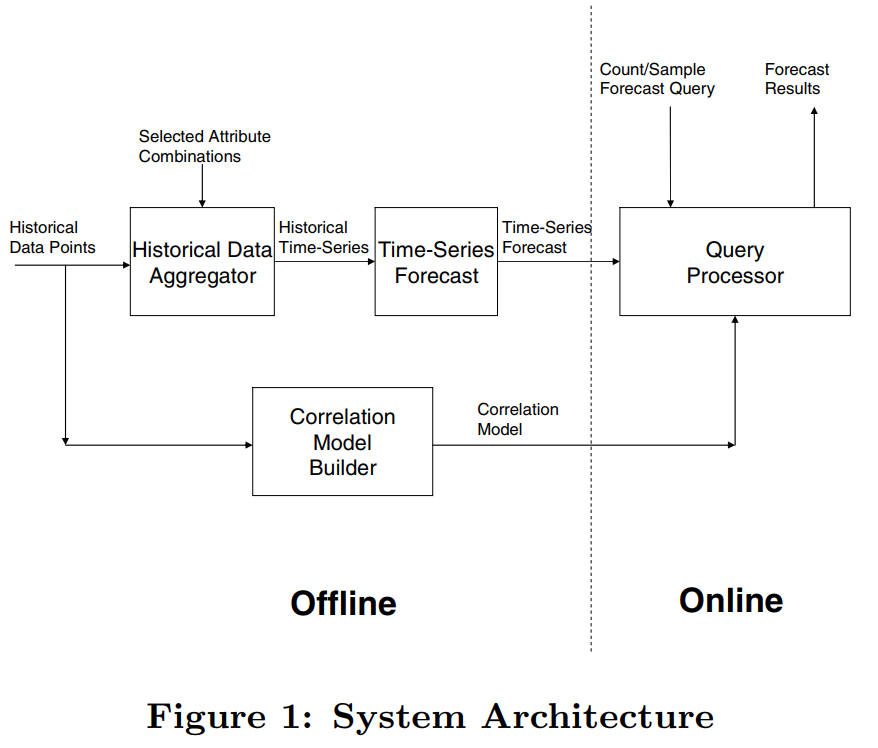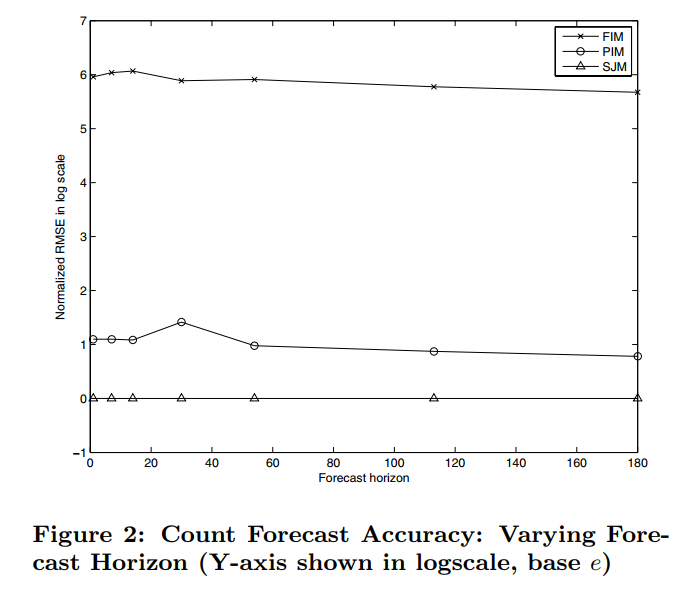Forecasting High-Dimensional Data》 是 Yahoo! 一篇关于流量预估的论文。在合约广告中，需要提前预估某个定向下的流量情况，从而进行合理的售卖和分配。但是由于定向的组合非常多（广告主的多样的需求导致的），而工程上不允许为每个可能的定向预估其流量，因此这篇论文提出了先预估一些基本定向的流量，然后通过 correlation model 从基本定向的流量计算出各种定向下的流量情况，具有较强的工程性，也是之前提到的文章 《Budget Pacing for Targeted Online Advertisements at LinkedIn》 中采用的流量预估方法。

## 系统总览

（1）送入到 Historical Data Aggregator 中对某些有代表性的 Selected Attribute Combinations 进行历史统计，并通过模型进行时间序列的预估，这里的时间序列预估使用了 SARIMA 模型
（2）用于构建 Correlation Model，并与上面若干 Selected Attribute Combinations 的预估结果共同对在线的 query 返回预估值## Correlation Model

1. 选择 $Q$ 中某个 base query $Q_k$, 使得 $q \subseteq Q_k$
2. 计算 $Q_k$ 在未来时间 $T$ 内的流量，记为 $B(Q_k, T)$
3. 计算 $q$ 在 $Q_k$ 中出现的概率，记为 $R(q|Q_k)$
4. 返回 $q$ 的预估结果为 $B(Q_k, T) \times R(q|Q_k)$

Correlation Model 所做的事情就是第3步：计算比例。

### Full Independence Model (FIM)

FIM 实际上就是个 Naive Bayes，假设了每个 attribute 是相互独立的，也就是

$$R(Gender = male \wedge Age < 30|Q_k) = R(Gender = male|Q_k) \times R(Age < 30|Q_k)$$

$$R(a_i|Q_k) = \frac{|a_i \in (Q_k \wedge P_t)|}{|Q_k \wedge P_t|}$$

### Partwise Independence Model (PIM)

$$R(Gender = male \wedge Age < 30 \wedge Incom > 10000|Q_k) = R(Gender = male|Q_k) \times R(Age < 30 \wedge Incom > 10000|Q_k)$$, 而比例的计算公式也跟上面的类似

### Sampling-based Joint Model(SJM)

$$R(q|Q_k) = \frac{n}{|Q_k \cap S|}$$

## 实验效果

### 评估指标

$$APE = \frac{|F-A|}{A}$$

APE 针对的是单个 query，但是往往希望的是验证一系列 query 的效果，其指标为 Root Mean Square Error (RMSE)，定义如下, $w_q$ 表示每个 query 的权重，这个值被设为 query 在过去两年的合约中出现的次数

$$RMSE = \sqrt{\frac{\sum_{q \in Q}w_qAPE^2(q)}{\sum_{q \in Q}w_q}}$$

### 效果对比